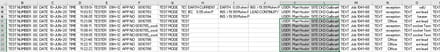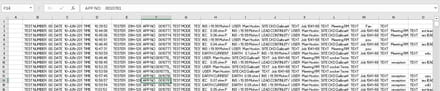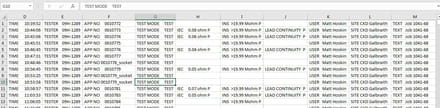# Convert Single Column to Rows, into Matching Rows

Solved/Closed
alansanderson Posts 3 Registration date Sunday June 12, 2016 Status Member Last seen June 19, 2016 - Jun 14, 2016 at 10:36 AM
TrowaD Posts 2921 Registration date Sunday September 12, 2010 Status Moderator Last seen January 16, 2023 - Jun 20, 2016 at 11:45 AM
Hi, I would really appreciate any help with an issue I currently have.

I have a file which downloads as ASCII (text), and when opened in excel gives a creates one column of data as per below. I want to convert this data to rows, using the line break as a new row, however the issue I have that the text data is not symmetric, so when I convert to rows the columns do not line up.

TEST NUMBER 0001
DATE 10-JUN-2016
TIME 10:39:52
TESTER 09H-1289
APP NO 0010772
TEST MODE TEST
INS >19.99 Mohm P
USER Matt Hoskin
SITE CKD Galbraith
TEXT Job 1041-68
TEXT Meeting RM
TEXT Fan
TEXT

TEST NUMBER 0002
DATE 10-JUN-2016
TIME 10:44:06
TESTER 09H-1289
APP NO 0010773
TEST MODE TEST
IEC 0.08 ohm P
INS >19.99 Mohm P
USER Matt Hoskin
SITE CKD Galbraith
TEXT Job 1041-68
TEXT Meeting RM
TEXT

TEST NUMBER 0003
DATE 10-JUN-2016
TIME 10:45:06
TESTER 09H-1289
APP NO 0010774
TEST MODE TEST
IEC 0.06 ohm P
INS >19.99 Mohm P
USER Matt Hoskin
SITE CKD Galbraith
TEXT Job 1041-68
TEXT Meeting RM
TEXT

What I am trying to achieve, as per the screen shot, so the data falls into the correct column and each block represents a new row.I have quite a lot of the files, and was trying to create a Macro / Script to help automate this, but can't get it to work, if anybody has any idea's I would be eternally grateful, as otherwise I am going to be cutting and pasting for the next 100 years :)

Related:

## 3 responses

TrowaD Posts 2921 Registration date Sunday September 12, 2010 Status Moderator Last seen January 16, 2023 549
Jun 16, 2016 at 11:29 AM
Hi Alansanderson,

I hope you realise that eternally is a very long time ... :)

But if you are ready for that kind of commitment, try the following code:
```Sub RunMe()
Dim x, lRow As Long

x = 1
Do
lRow = Cells(x, "A").End(xlDown).Row
Range(Cells(x, "A"), Cells(lRow, "A")).Copy
Range("B" & Rows.Count).End(xlUp).Offset(1, 0).PasteSpecial Transpose:=True
x = lRow + 2
Loop Until Cells(x, "A").Value = vbNullString

Application.CutCopyMode = False
End Sub```

Best regards,
Trowa
alansanderson Posts 3 Registration date Sunday June 12, 2016 Status Member Last seen June 19, 2016
Jun 17, 2016 at 02:14 AM
Thanks Trowa,

Trust me, after spending about 8 hours copying and pasting, I know eternally is a long long time :)

This almost works, it transposes column A into rows great, however it doesn't line up the columns. Column B to G is perfect, but it then goes a bit haywire in columns G to P, Essentially I am trying to get the columns to align and this is the bit I just cant get to work.Now I know this is because the data that makes up row two, has more information, therefore more rows in the data equals more columns, but I was trying to get it to leave column blank if no data is in the block for that row.However the issue is compounded as there is no regular pattern to the data.

Thanks for trying Trowa, this piece of code is neater and more efficient than the transpose I had written, never been great with VB, so I will be using this to transpose from now on.

Given the length of time I have spent cutting and pasting on just one file, I will be eternally, eternally, eternally grateful to anybody who can create a solution as I have 300 of these files to convert.

Alan
alansanderson Posts 3 Registration date Sunday June 12, 2016 Status Member Last seen June 19, 2016
Jun 19, 2016 at 05:53 PM
Thanks for the help, really appreciate it.

I have managed to find a way to do it, probably not the neatest or most efficient but it works :)

Basically I used a match to pull out certain field, then removed some fields and re-ran the transpose to get the text fields as I couldn't find a simple solution to the match.

As I said not neat and tidy, and still requires a number of steps, with very little error handling but for a newbie I'm happy it does what I need it to do.

`Sub Convert_to_Rows()Sheets("Sheet1").Select    Range("A1").SelectDim x, lRow As Longx = 1Do    lRow = Cells(x, "A").End(xlDown).Row    Range(Cells(x, "A"), Cells(lRow, "A")).Copy    Range("B" & Rows.Count).End(xlUp).Offset(1, 0).PasteSpecial Transpose:=True    x = lRow + 2Loop Until Cells(x, "A").Value = vbNullStringApplication.CutCopyMode = False   'copy to sheet 6       Sheets("Sheet1").Select    Columns("A:A").Select    Selection.Copy    Sheets("Sheet6").Select    Columns("A:A").Select    Selection.Insert Shift:=xlToRight    Sheets("Menu").Select' Delete A from sheet 1Sheets("Sheet1").Select    Columns("A:A").Select    Selection.Delete Shift:=xlToLeft            Sheets("Menu").SelectEnd SubSub Create_Raw_Data()    Sheets("Sheet1").Columns("A:F").Copy Sheets("Raw").Range("A1")        Dim LR As LongLR = ActiveSheet.UsedRange.Rows.Count + 1Sheets("Raw").Select    Dim ws As Worksheet    Dim lRow As Long    '~~> Change this to the relevant sheet    Set ws = ThisWorkbook.Sheets("Raw")    With ws        '~~> Find last row in Col A        lRow = .Range("A" & .Rows.Count).End(xlUp).Row        '~~> Enter the formula in one go        .Range("G1:G" & lRow).FormulaR1C1 = "=IFERROR(INDEX(Sheet1!R, MATCH("" Site*"", Sheet1!R, 0)),"""")   "        .Range("H1:H" & lRow).FormulaR1C1 = "=IFERROR(INDEX(Sheet1!R, MATCH("" User*"", Sheet1!R, 0)),"""")   "        .Range("I1:I" & lRow).FormulaR1C1 = "=IFERROR(INDEX(Sheet1!R, MATCH("" INS*"", Sheet1!R, 0)),"""")    "        .Range("J1:J" & lRow).FormulaR1C1 = "=IFERROR(INDEX(Sheet1!R, MATCH("" IEC*"", Sheet1!R, 0)),"""")    "        .Range("K1:K" & lRow).FormulaR1C1 = "=IFERROR(INDEX(Sheet1!R, MATCH("" EARTH C*"", Sheet1!R, 0)),"""")    "        .Range("L1:L" & lRow).FormulaR1C1 = "=IFERROR(INDEX(Sheet1!R, MATCH("" EARTH  *"", Sheet1!R, 0)),"""")    "        .Range("M1:M" & lRow).FormulaR1C1 = "=IFERROR(INDEX(Sheet1!R, MATCH("" Lead*"", Sheet1!R, 0)),"""")    "    End With        Sheets("Menu").Select        End Sub        Sub Delete_Extra_Data()'Delete all INS, Earth & Lead then convert to rowsSheets("Sheet6").SelectWith ActiveSheet    .AutoFilterMode = False    With Range("a1", Range("a" & Rows.Count).End(xlUp))        .AutoFilter 1, " INS*"        On Error Resume Next        .Offset(1).SpecialCells(12).EntireRow.Delete    End With    .AutoFilterMode = FalseEnd WithWith ActiveSheet    .AutoFilterMode = False    With Range("a1", Range("a" & Rows.Count).End(xlUp))        .AutoFilter 1, " LEAD*"        On Error Resume Next        .Offset(1).SpecialCells(12).EntireRow.Delete    End With    .AutoFilterMode = FalseEnd WithWith ActiveSheet    .AutoFilterMode = False    With Range("a1", Range("a" & Rows.Count).End(xlUp))        .AutoFilter 1, " IEC*"        On Error Resume Next        .Offset(1).SpecialCells(12).EntireRow.Delete    End With    .AutoFilterMode = FalseEnd WithWith ActiveSheet    .AutoFilterMode = False    With Range("a1", Range("a" & Rows.Count).End(xlUp))        .AutoFilter 1, " EARTH*"        On Error Resume Next        .Offset(1).SpecialCells(12).EntireRow.Delete    End With    .AutoFilterMode = FalseEnd WithSheets("Sheet6").Select    Range("A1").SelectDim x, lRow As Longx = 1Do    lRow = Cells(x, "A").End(xlDown).Row    Range(Cells(x, "A"), Cells(lRow, "A")).Copy    Range("B" & Rows.Count).End(xlUp).Offset(1, 0).PasteSpecial Transpose:=True    x = lRow + 2Loop Until Cells(x, "A").Value = vbNullStringApplication.CutCopyMode = False' Delete A from sheet 1Sheets("Sheet6").Select    Columns("A:A").Select    Selection.Delete Shift:=xlToLeft            Sheets("Menu").SelectEnd SubSub Format_Results()If MsgBox("Are All The States Green, if not the results will not process, please cancel and do the step which is red", vbYesNo + vbQuestion) = vbNo Then    Exit Sub  End IfDim ws As Worksheet    Dim lRow As Long    '~~> Change this to the relevant sheet    Set ws = ThisWorkbook.Sheets("Sheet3")    With ws        '~~> Enter the formula in one go        .Range("A6:A3026").FormulaR1C1 = "=RIGHT(Raw!R[-4]C,LEN(Raw!R[-4]C)-12)"        .Range("B6:B3026").FormulaR1C1 = "=RIGHT(Raw!R[-4]C,LEN(Raw!R[-4]C)-6)"        .Range("C6:C3026").FormulaR1C1 = "=RIGHT(Raw!R[-4]C,LEN(Raw!R[-4]C)-6)"        .Range("D6:D3026").FormulaR1C1 = "=RIGHT(Raw!R[-4]C,LEN(Raw!R[-4]C)-8)"        .Range("E6:E3026").FormulaR1C1 = "=RIGHT(Raw!R[-4]C,LEN(Raw!R[-4]C)-6)"        .Range("F6:F3026").FormulaR1C1 = "=IF(ISERROR(RIGHT(Raw!R[-4]C,LEN(Raw!R[-4]C)-6)),"""",(RIGHT(Raw!R[-4]C,LEN(Raw!R[-4]C)-6)) )"        .Range("G6:G3026").FormulaR1C1 = "=IF(ISERROR(RIGHT(Raw!R[-4]C,LEN(Raw!R[-4]C)-6)),"""",(RIGHT(Raw!R[-4]C,LEN(Raw!R[-4]C)-6)) )"        .Range("H6:H3026").FormulaR1C1 = "=IF(ISERROR(RIGHT(Raw!R[-4]C,LEN(Raw!R[-4]C)-6)),"""",(RIGHT(Raw!R[-4]C,LEN(Raw!R[-4]C)-6)) )"        .Range("I6:I3026").FormulaR1C1 = "=IF(ISERROR(RIGHT(Raw!R[-4]C,LEN(Raw!R[-4]C)-17)),"""",(RIGHT(Raw!R[-4]C,LEN(Raw!R[-4]C)-17)) )"        .Range("J6:J3026").FormulaR1C1 = "=RIGHT(Sheet6!R[-4]C,LEN(Sheet6!R[-4]C)-5)"        .Range("K6:K3026").FormulaR1C1 = "=RIGHT(Sheet6!R[-4]C[-1],LEN(Sheet6!R[-4]C[-1])-9)"        .Range("L6:L3026").FormulaR1C1 = "=IF(ISERROR(RIGHT(Sheet6!R[-4]C,LEN(Sheet6!R[-4]C)-6)),"""",(RIGHT(Sheet6!R[-4]C,LEN(Sheet6!R[-4]C)-6)) )"            End With        Sheets("Sheet3").Select    Range("A6:L3026").Select        With Selection        .HorizontalAlignment = xlGeneral        .VerticalAlignment = xlBottom        .WrapText = False        .Orientation = 0        .AddIndent = False        .IndentLevel = 0        .ShrinkToFit = False        .ReadingOrder = xlContext        .MergeCells = False    End With    With Selection        .HorizontalAlignment = xlRight        .VerticalAlignment = xlBottom        .WrapText = False        .Orientation = 0        .AddIndent = False        .IndentLevel = 0        .ShrinkToFit = False        .ReadingOrder = xlContext        .MergeCells = False    End With         Sheets("Sheet3").Select     ActiveSheet.Range("\$A\$5:\$L\$3026").AutoFilter Field:=1, Criteria1:="*", Operator:=xlFilterValues                End Sub`
TrowaD Posts 2921 Registration date Sunday September 12, 2010 Status Moderator Last seen January 16, 2023 549
Jun 20, 2016 at 11:45 AM
Hi Alan,

Good to see you worked it out.

I missed the aligning columns part.

If you are up to it, you can try this code:
```Sub RunMe()
Dim x, y, lRow As Long

x = 1
Do
lRow = Cells(x, "A").End(xlDown).Row
Range(Cells(x, "A"), Cells(lRow, "A")).Copy
Range("B" & Rows.Count).End(xlUp).Offset(1, 0).PasteSpecial Transpose:=True
x = lRow + 2
Loop Until Cells(x, "A").Value = vbNullString

Application.CutCopyMode = False

x = 2
Do Until IsEmpty(Cells(x, "H"))
If Left(Cells(x, "H"), 3) <> "IEC" Then
Range(Cells(x, "H"), Cells(x, "Z")).Cut Cells(x, "I")
End If
x = x + 1
Loop

x = 2
Do Until IsEmpty(Cells(x, "I"))
If Left(Cells(x, "I"), 3) <> "INS" Then
Range(Cells(x, "I"), Cells(x, "Z")).Cut Cells(x, "J")
End If
x = x + 1
Loop

x = 2
Do Until IsEmpty(Cells(x, "J"))
If Left(Cells(x, "J"), 4) <> "LEAD" Then
Range(Cells(x, "J"), Cells(x, "Z")).Cut Cells(x, "K")
End If
x = x + 1
Loop

End Sub```

It works for your sample data, but I don't know how the rest of your data looks like.

Bummer, I guess I missed my shot at eternal gratitude ;)

Best regards,
Trowa